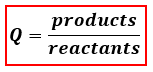# Problem: Consider the hypothetical reaction below3 A (g) + B (g) ↔ 2 C (g)           Kc = 1.5 x 10-3Which of the following statements is correct if the initial concentrations are [A] = 0.85 M, [B] = 0.36 M, and [C] = 0.005 M.A. At equilibrium, the amount of C will increase.B. At equilibrium, the amount of A will increase.C. At equilibrium, the amount of B will increase and C will increase.D. At equilibrium, the amount of A will increase and B will decrease.E. The reaction is at equilibrium.

###### FREE Expert Solution
85% (463 ratings)
###### FREE Expert Solution

We are being asked what would happen to the equilibrium reaction given the initial concentrations of the species. We will use the reaction quotient, Q, to determine if a chemical reaction would be at equilibrium or not. The formula for Q is:Depending on if Q is greater than or less than K our reaction will shift to attain equilibrium by reaching the equilibrium constant K:

85% (463 ratings)###### Problem Details

Consider the hypothetical reaction below
3 A (g) + B (g) ↔ 2 C (g)           Kc = 1.5 x 10-3

Which of the following statements is correct if the initial concentrations are [A] = 0.85 M, [B] = 0.36 M, and [C] = 0.005 M.

A. At equilibrium, the amount of C will increase.

B. At equilibrium, the amount of A will increase.

C. At equilibrium, the amount of B will increase and C will increase.

D. At equilibrium, the amount of A will increase and B will decrease.

E. The reaction is at equilibrium.